Theory and Modern Applications

Convergence and stability of implicit compensated Euler method for stochastic differential equations with Poisson random measure

Abstract

In this paper, an implicit compensated Euler method is introduced for stochastic differential equations with Poisson random measure. A convergence theorem is proved to show that the method obtains a strong order 0.5. After exploiting the conditions of exponential mean-square stability of such equations, the implicit compensated Euler method is proved to share the same stability for any step size. Numerical examples indicate the performance of the convergence and stability.

1 Introduction

In finance and economics, in order to obtain the dynamics observed, it is important to model the impact of event-driven uncertainty. Events such as corporate defaults, operational failures, market crashes or central bank announcements lead to the introduction of stochastic differential equations (SDEs) driven by random measure; see [1, 2] since such equations were initiated by Merton .

Since only explicit solutions of a small class of SDEs with Poisson random measure can be obtained, one needs, in general, discrete time approximations which can be divided into strong approximations and weak approximations. Strong approximations provide pathwise approximations, while weak approximations are appropriate for problems such as derivative pricing or the evaluation of risk measures and expected utilities.

We give an overview of the existing literature on the strong approximations of SDEs with Poisson random measure. Early, in , Platen gave a convergence theorem for strong approximations of any given order $\gamma \in \left\{0.5,1,1.5,\dots \right\}$ and originally introduced the so-called jump-adapted schemes which were based on time discretizations that included all the jump times. Moreover, by using an order 1.5 scheme for approximating the diffusion part, Kloeden and Platen (see ) obtained the jump-adapted order 1.5 strong scheme, and they also constructed the derivative free or implicit jump-adapted schemes with desired order of strong convergence. In , for the specific case of pure jump SDEs, the strong order of convergence of Taylor schemes was established under weaker conditions than those currently known in the literature. Recently, Bruti-Liberati and Platen [5, 7] have presented the drift-implicit schemes which achieve strong order $\gamma \in \left\{0.5,1\right\}$. Mao  deals with the convergence of numerical solutions for variable delay differential equations driven by Poisson random measure. In , the improved Runge-Kutta methods have been presented to improve the accuracy behavior of problems with small noise for SDEs with Poisson random measure. Weak approximations can be seen in [7, 10, 11].

As for the stability of SDEs with Poisson random measure, only limited results have been presented in currently known literature. Li, Dong and Situ  have shown that the almost sure asymptotic stability of linear SDEs with Poisson random measure depends on the negative Lyapunov exponential functions. Using Liapunov’s method, Swishchuk and Kazmerchuk  have presented the stability of a trivial solution of semi-linear stochastic delay differential equations with Markovian switchings and with Poisson bifurcations.

However, to the best of our knowledge, little has been presented about the stability of numerical methods to SDEs with Poisson random measure. The main contribution in our work shows that the implicit compensated Euler method shares exponential mean-square stability for any step size Δt with SDEs with Poisson random measure; that is, the method can preserve exponential mean-square stability without any restrictions on a step size. To some extent, our numerical experiments demonstrate that the stability behavior of the implicit compensated Euler method is not influenced by an increasing step size, while the Euler method and drift-implicit Euler method can only preserve stability for restricted step sizes. Our work is motivated by [57, 14]. In [5, 7], the drift-implicit Euler method of order 0.5 was introduced to SDEs with Poisson random measure and its convergence was considered. In , Euler method was presented to SDEs with Poisson random measure. In , the stability was analyzed for the compensated split-step backward (CSSBE) method and split-step backward Euler (SSBE) method to SDEs with Poisson process.

Our work is organized as follows. In Section 1, an implicit compensated Euler method is introduced to SDEs with Poisson random measure. In Section 2, a convergence theorem of the method is proved by four lemmas under the Lipschitz conditions and the linear growth conditions. In Section 3, the exponential mean-square stability of such an equation is analyzed. Subsequently, the stability of the implicit compensated Euler method to such an equation is presented in the last theorem under the one-sided Lipschitz conditions. In Section 4, some numerical experiments show the performance of the convergence and stability. Finally, a concluding remark is given.

1.1 Problem’s setting

Throughout this paper, unless otherwise specified, we use the following notations. Let ${u}_{1}\vee {u}_{2}=max\left\{{u}_{1},{u}_{2}\right\}$. Let $|\cdot |$ and $〈\cdot ,\cdot 〉$ be the Euclidean norm and the inner product of vectors in ${\mathbf{R}}^{d}$, $d\in \mathbf{N}$. If A is a vector or matrix, its transpose is denoted by ${A}^{T}$. If A is a matrix, its trace norm is denoted by $|A|=\sqrt{trace\left({A}^{T}A\right)}$. Let ${L}_{{\mathcal{F}}_{0}}^{2}\left(\mathrm{\Omega };{\mathbf{R}}^{d}\right)$ denote the family of ${\mathbf{R}}^{d}$-valued ${\mathcal{F}}_{0}$-measurable random variables ξ with $\mathbf{E}{|\xi |}^{2}<\mathrm{\infty }$. ${C}^{1}\left({\mathbf{R}}^{d};{\mathbf{R}}^{d}\right)$ denotes the family of continuously differentiable ${\mathbf{R}}^{d}$-valued functions defined on ${\mathbf{R}}^{d}$.

The following d-dimensional stochastic differential equation with Poisson random measure is considered in our paper:

$dx\left(t\right)=a\left(x\left(t-\right)\right)\phantom{\rule{0.2em}{0ex}}dt+b\left(x\left(t-\right)\right)\phantom{\rule{0.2em}{0ex}}dW\left(t\right)+{\int }_{\epsilon }c\left(x\left(t-\right),v\right){p}_{\varphi }\left(dv×dt\right),\phantom{\rule{1em}{0ex}}t>0$
(1.1)

with initial condition $x\left(0-\right)=x\left(0\right)={x}_{0}\in {L}_{{\mathcal{F}}_{0}}^{2}\left(\mathrm{\Omega };{\mathbf{R}}^{d}\right)$, where $x\left(t-\right)$ denotes ${lim}_{s\to t-}x\left(s\right)$.

The randomness in this equation is generated by the following (see ). An m-dimensional Wiener process $W=\left\{W\left(t\right)={\left({W}^{1}\left(t\right),\dots ,{W}^{m}\left(t\right)\right)}^{T}\right\}$ with independent scalar components is defined on a filtered probability space $\left({\mathrm{\Omega }}^{W},{\mathcal{F}}^{W},{\left({\mathcal{F}}_{t}^{W}\right)}_{t\ge 0},{\mathbf{P}}^{W}\right)$. A Poisson random measure ${p}_{\varphi }\left(\omega ,dv×dt\right)$ is on ${\mathrm{\Omega }}^{J}×\epsilon ×\left[0,\mathrm{\infty }\right)$, where $\epsilon \subseteq {\mathbf{R}}^{r}\setminus \left\{0\right\}$, with $r\in \mathbf{N}$, and its deterministic compensated measure $\varphi \left(dv\right)\phantom{\rule{0.2em}{0ex}}dt=\lambda f\left(v\right)\phantom{\rule{0.2em}{0ex}}dv\phantom{\rule{0.2em}{0ex}}dt$. $f\left(v\right)$ is a probability density, and we require finite intensity $\lambda =\varphi \left(\epsilon \right)<\mathrm{\infty }$. The Poisson random measure is defined on a filtered probability space $\left({\mathrm{\Omega }}^{J},{\mathcal{F}}^{J},{\left({\mathcal{F}}_{t}^{J}\right)}_{t\ge 0},{\mathbf{P}}^{J}\right)$. As a consequence, the process x is defined on a product space $\left(\mathrm{\Omega },\mathcal{F},{\left({\mathcal{F}}_{t}\right)}_{t\ge 0},\mathbf{P}\right)$, where $\mathrm{\Omega }={\mathrm{\Omega }}^{W}×{\mathrm{\Omega }}^{J}$, $\mathcal{F}={\mathcal{F}}^{W}×{\mathcal{F}}^{J}$, ${\left({\mathcal{F}}_{t}\right)}_{t\ge 0}={\left({\mathcal{F}}_{t}^{W}\right)}_{t\ge 0}×{\left({\mathcal{F}}_{t}^{J}\right)}_{t\ge 0}$, $\mathbf{P}={\mathbf{P}}^{W}×{\mathbf{P}}^{J}$ and ${\mathcal{F}}_{0}$ contains all P-null sets. The Wiener process and the Poisson random measure are mutually independent.

The drift coefficient $a:{\mathbf{R}}^{d}\to {\mathbf{R}}^{d}$, the diffusion coefficient $b:{\mathbf{R}}^{d}\to {\mathbf{R}}^{d×m}$ and the jump coefficient $c:{\mathbf{R}}^{d}×\epsilon \to {\mathbf{R}}^{d}$ are usually assumed to be Borel measurable functions and the coefficients satisfy the Lipschitz conditions

${|a\left(x\right)-a\left(y\right)|}^{2}\vee {|b\left(x\right)-b\left(y\right)|}^{2}\vee {\int }_{\epsilon }{|c\left(x,v\right)-c\left(y,v\right)|}^{2}\varphi \left(dv\right)\le {K}_{1}{|x-y|}^{2}$
(1.2)

for every $x,y\in {\mathbf{R}}^{d}$ and ${K}_{1}>0$, and the linear growth conditions

${|a\left(x\right)|}^{2}\vee {|b\left(x\right)|}^{2}\vee {\int }_{\epsilon }{|c\left(x,v\right)|}^{2}\varphi \left(dv\right)\le {K}_{2}\left(1+{|x|}^{2}\right)$
(1.3)

for all $x\in {\mathbf{R}}^{d}$ and ${K}_{2}=max\left\{2{K}_{1},2{|a\left(0\right)|}^{2},2{|b\left(0\right)|}^{2},2{\int }_{\epsilon }{|c\left(0,v\right)|}^{2}\varphi \left(dv\right)\right\}$, where

${|a\left(0\right)|}^{2}+{|b\left(0\right)|}^{2}+{\int }_{\epsilon }{|c\left(0,v\right)|}^{2}\varphi \left(dv\right)\le L,\phantom{\rule{1em}{0ex}}L>0.$

A unique strong solution of the equation (1.1) exists under the conditions (1.2) and (1.3); see [15, 16].

1.2 Implicit compensated Euler method

It is convenient to rewrite the equation (1.1) in terms of the compensated Poisson measure (see )

${\stackrel{˜}{p}}_{\varphi }\left(dv×dt\right):={p}_{\varphi }\left(dv×dt\right)-\varphi \left(dv\right)\phantom{\rule{0.2em}{0ex}}dt,$

as

$\begin{array}{rcl}dx\left(t\right)& =& \left(a\left(x\left(t-\right)\right)+{\int }_{\epsilon }c\left(x\left(t-\right),v\right)\varphi \left(dv\right)\right)\phantom{\rule{0.2em}{0ex}}dt+b\left(x\left(t-\right)\right)\phantom{\rule{0.2em}{0ex}}dW\left(t\right)\\ +{\int }_{\epsilon }c\left(x\left(t-\right),v\right){\stackrel{˜}{p}}_{\varphi }\left(dv×dt\right),\end{array}$
(1.4)

for $x\in {\mathbf{R}}^{d}$.

Given a step size $\mathrm{\Delta }t>0$, the implicit compensated Euler method applied to (1.1) computes approximation ${Y}_{n}\approx x\left({t}_{n}\right)$, where ${t}_{n}=n\mathrm{\Delta }t$, $n=0,1,\dots$ , by setting ${Y}_{0}={x}_{0}$ and forming

$\begin{array}{rcl}{Y}_{n+1}& =& {Y}_{n}+\left(a\left({Y}_{n+1}\right)+{\int }_{\epsilon }c\left({Y}_{n+1},v\right)\varphi \left(dv\right)\right)\mathrm{\Delta }t+b\left({Y}_{n}\right)\mathrm{\Delta }{W}_{n}\\ +{\int }_{{t}_{n}}^{{t}_{n+1}}{\int }_{\epsilon }c\left({Y}_{n},v\right){\stackrel{˜}{p}}_{\varphi }\left(dv×dt\right),\end{array}$
(1.5)

where $\mathrm{\Delta }{W}_{n}=W\left({t}_{n+1}\right)-W\left({t}_{n}\right)$.

The continuous-time implicit compensated Euler method is then defined by

$\begin{array}{rcl}\overline{Y}\left(t\right)& :=& {Y}_{0}+{\int }_{0}^{t}\left(a\left({Z}_{2}\left(s-\right)\right)+{\int }_{\epsilon }c\left({Z}_{2}\left(s-\right),v\right)\varphi \left(dv\right)\right)\phantom{\rule{0.2em}{0ex}}ds+{\int }_{0}^{t}b\left({Z}_{1}\left(s-\right)\right)\phantom{\rule{0.2em}{0ex}}dW\left(s\right)\\ +{\int }_{0}^{t}{\int }_{\epsilon }c\left({Z}_{1}\left(s-\right),v\right){\stackrel{˜}{p}}_{\varphi }\left(dv×ds\right),\end{array}$
(1.6)

where ${Z}_{1}\left(t\right)={Y}_{n}$, ${Z}_{2}\left(t\right)={Y}_{n+1}$ for $t\in \left[{t}_{n},{t}_{n+1}\right)$, $n=0,1,\dots$ .

Actually, as we see in , ${p}_{\varphi }=\left\{{p}_{\varphi }\left(t\right):={p}_{\varphi }\left(\epsilon ×\left[0,t\right]\right)\right\}$ is a process that counts the number of jumps until some given time. The Poisson random measure ${p}_{\varphi }\left(dv×dt\right)$ generates a sequence of pairs $\left\{\left({\tau }_{i},{\xi }_{i}\right),i\in \left\{1,2,\dots ,{p}_{\varphi }\left(T\right)\right\}\right\}$ for a given finite positive constant T if $\lambda <\mathrm{\infty }$. Here $\left\{{\tau }_{i}:\mathrm{\Omega }\to {\mathbf{R}}_{+},i\in \left\{1,2,\dots ,{p}_{\varphi }\left(T\right)\right\}\right\}$ is a sequence of increasing nonnegative random variables representing the jump times of a standard Poisson process with intensity λ, and $\left\{{\xi }_{i}:\mathrm{\Omega }\to \epsilon ,i\in \left\{1,2,\dots ,{p}_{\varphi }\left(T\right)\right\}\right\}$ is a sequence of independent identically distributed random variables, where ${\xi }_{i}$ is distributed according to $\varphi \left(dv\right)/\varphi \left(\epsilon \right)$. Then (1.5) can equivalently be of the following form:

$\begin{array}{rcl}{Y}_{n+1}& =& {Y}_{n}+\left(a\left({Y}_{n+1}\right)+{\int }_{\epsilon }c\left({Y}_{n+1},v\right)\varphi \left(dv\right)\right)\mathrm{\Delta }t+b\left({Y}_{n}\right)\mathrm{\Delta }{W}_{n}\\ -{\int }_{\epsilon }c\left({Y}_{n},v\right)\varphi \left(dv\right)\mathrm{\Delta }t+\sum _{i={p}_{\varphi }\left({t}_{n}\right)+1}^{{p}_{\varphi }\left({t}_{n+1}\right)}c\left({Y}_{n},{\xi }_{i}\right).\end{array}$
(1.7)

As the special case of (1.1), that is, $c\left(x,v\right)=c\left(x\right)$, the method (1.5) reduces to

${Y}_{n+1}={Y}_{n}+\left(a\left({Y}_{n+1}\right)+\lambda c\left({Y}_{n+1}\right)\right)\mathrm{\Delta }t+b\left({Y}_{n}\right)\mathrm{\Delta }{W}_{n}-\lambda c\left({Y}_{n}\right)\mathrm{\Delta }t+c\left({Y}_{n}\right)\mathrm{\Delta }{p}_{n},$

where $\mathrm{\Delta }{p}_{n}={p}_{\varphi }\left({t}_{n+1}\right)-{p}_{\varphi }\left({t}_{n}\right)$ is a Poisson distributed random variable with mean $\lambda \mathrm{\Delta }t$.

2 Strong convergence of implicit compensated Euler method

In this section, we present a convergence theorem of the implicit compensated Euler method (1.5) to the SDE with Poisson random measure (1.1) over a finite time interval $\left[0,T\right]$ under the Lipschitz conditions and the linear growth conditions, where T is a constant and $\mathrm{\Delta }t=T/N$, $N\in \mathbf{N}$. At the beginning, we give four lemmas.

The first lemma demonstrates the existence of a solution to the implicit compensated Euler method (1.5).

Lemma 2.1 Under the Lipschitz conditions (1.2), if $\left(\sqrt{{K}_{1}}+\sqrt{\lambda {K}_{1}}\right)\mathrm{\Delta }t<1$, then the equation for the implicit compensated Euler method (1.5) can be solved uniquely for ${Y}_{n+1}$ given ${Y}_{n}$, with probability $one$.

Proof Writing (1.5) as ${Y}_{n+1}=F\left({Y}_{n+1}\right)$ and then using the Cauchy-Schwarz inequality and (1.2), we have

$\begin{array}{rcl}|F\left({u}_{1}\right)-F\left({u}_{2}\right)|& =& |a\left({u}_{1}\right)-a\left({u}_{2}\right)+{\int }_{\epsilon }c\left({u}_{1},v\right)\varphi \left(dv\right)-{\int }_{\epsilon }c\left({u}_{2},v\right)\varphi \left(dv\right)|\mathrm{\Delta }t\\ \le & |a\left({u}_{1}\right)-a\left({u}_{2}\right)|\mathrm{\Delta }t+{\left({\int }_{\epsilon }{1}^{2}\varphi \left(dv\right){\int }_{\epsilon }{|c\left({u}_{1},v\right)-c\left({u}_{2},v\right)|}^{2}\varphi \left(dv\right)\right)}^{1/2}\mathrm{\Delta }t\\ \le & \left(\sqrt{{K}_{1}}+\sqrt{\lambda {K}_{1}}\right)\mathrm{\Delta }t|{u}_{1}-{u}_{2}|,\end{array}$

for ${u}_{1},{u}_{2}\in {\mathbf{R}}^{d}$. Hence, the result follows from the classical Banach contraction mapping theorem. □

The second lemma shows that the discrete implicit compensated Euler method (1.5) of the equation (1.1) has bounded second moments.

Lemma 2.2 Under the linear growth conditions (1.3), there exists $\mathrm{\Delta }{t}_{0}>0$ such that for all $0<\mathrm{\Delta }t<\mathrm{\Delta }{t}_{0}<1/\left(1+2{K}_{2}+2\lambda {K}_{2}\right)$,

(2.1)

where ${C}_{1}$ is a constant independent of Δt.

Proof It follows from (1.5) that

$\begin{array}{c}{|{Y}_{n+1}-\left(a\left({Y}_{n+1}\right)+{\int }_{\epsilon }c\left({Y}_{n+1},v\right)\varphi \left(dv\right)\right)\mathrm{\Delta }t|}^{2}\hfill \\ \phantom{\rule{1em}{0ex}}={|{Y}_{n}+b\left({Y}_{n}\right)\mathrm{\Delta }{W}_{n}+{\int }_{{t}_{n}}^{{t}_{n+1}}{\int }_{\epsilon }c\left({Y}_{n},v\right){\stackrel{˜}{p}}_{\varphi }\left(dv×dt\right)|}^{2}.\hfill \end{array}$

Thus, by taking expectations and using the martingale properties of $d{W}_{n}$ and ${\stackrel{˜}{p}}_{\varphi }\left(dv×dt\right)$, we have

$\begin{array}{rcl}\mathbf{E}{|{Y}_{n+1}|}^{2}& \le & 2\mathrm{\Delta }t\mathbf{E}〈{Y}_{n+1},a\left({Y}_{n+1}\right)+{\int }_{\epsilon }c\left({Y}_{n+1},v\right)\varphi \left(dv\right)〉+\mathbf{E}{|{Y}_{n}|}^{2}\\ +\mathrm{\Delta }t\mathbf{E}{|b\left({Y}_{n}\right)|}^{2}+\mathrm{E}{\int }_{{t}_{n}}^{{t}_{n+1}}{\int }_{\epsilon }{|c\left({Y}_{n},v\right)|}^{2}\varphi \left(dv\right)\phantom{\rule{0.2em}{0ex}}dt.\end{array}$
(2.2)

Now, using the inequalities $〈u,v〉\le |u||v|\le {\left(\frac{|u|+|v|}{2}\right)}^{2}\le \frac{{|u|}^{2}+{|v|}^{2}}{2}$ for $u,v\in {\mathbf{R}}^{d}$, the Cauchy-Schwarz inequality and the linear growth conditions (1.3), we can obtain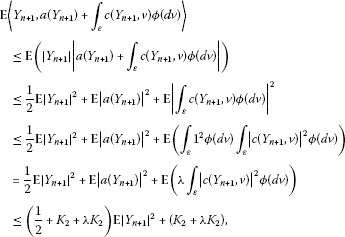(2.3)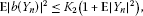(2.4)

and

$\mathbf{E}{\int }_{{t}_{n}}^{{t}_{n+1}}{\int }_{\epsilon }{|c\left({Y}_{n},v\right)|}^{2}\varphi \left(dv\right)\phantom{\rule{0.2em}{0ex}}dt\le {K}_{2}\mathrm{\Delta }t\left(1+\mathbf{E}{|{Y}_{n}|}^{2}\right).$
(2.5)

So, by substituting (2.3), (2.4) and (2.5) into (2.2) and then choosing any $\mathrm{\Delta }{t}_{0}\in \left(0,1/\left(1+2{K}_{2}+2\lambda {K}_{2}\right)\right)$, we get

$\begin{array}{rcl}\mathbf{E}{|{Y}_{n+1}|}^{2}& \le & \mathbf{E}{|{Y}_{n}|}^{2}+\frac{1+4{K}_{2}+2\lambda {K}_{2}}{1-\mathrm{\Delta }{t}_{0}\left(1+2{K}_{2}+2\lambda {K}_{2}\right)}\mathrm{\Delta }t\mathbf{E}{|{Y}_{n}|}^{2}\\ +\frac{4{K}_{2}+2\lambda {K}_{2}}{1-\mathrm{\Delta }{t}_{0}\left(1+2{K}_{2}+2\lambda {K}_{2}\right)}\mathrm{\Delta }t\end{array}$
(2.6)

for all $\mathrm{\Delta }t\in \left(0,\mathrm{\Delta }{t}_{0}\right)$. Summing the inequality (2.6) from 0 to $n\in \mathbf{N}$ when $n\mathrm{\Delta }t\le T$, we find

$\begin{array}{rcl}\mathbf{E}{|{Y}_{n}|}^{2}& \le & \mathbf{E}{|{Y}_{0}|}^{2}+\frac{1+4{K}_{2}+2\lambda {K}_{2}}{1-\mathrm{\Delta }{t}_{0}\left(1+2{K}_{2}+2\lambda {K}_{2}\right)}\mathrm{\Delta }t\sum _{i=0}^{n-1}\mathbf{E}{|{Y}_{i}|}^{2}\\ +\frac{4{K}_{2}+2\lambda {K}_{2}}{1-\mathrm{\Delta }{t}_{0}\left(1+2{K}_{2}+2\lambda {K}_{2}\right)}T,\end{array}$

which yields the following result by the discrete-type Gronwall inequality (see ) and $n\mathrm{\Delta }t\le T$

$\mathbf{E}{|{Y}_{n}|}^{2}\le \left(\mathbf{E}{|{Y}_{0}|}^{2}+\frac{4{K}_{2}T+2\lambda {K}_{2}T}{1-\mathrm{\Delta }{t}_{0}\left(1+2{K}_{2}+2\lambda {K}_{2}\right)}\right)exp\left(\frac{T+4{K}_{2}T+2\lambda {K}_{2}T}{1-\mathrm{\Delta }{t}_{0}\left(1+2{K}_{2}+2\lambda {K}_{2}\right)}\right).$

So, we have the result (2.1), where

$\begin{array}{rcl}{C}_{1}& =& max\left\{exp\left(\frac{\left(1+4{K}_{2}+2\lambda {K}_{2}\right)T}{1-\mathrm{\Delta }{t}_{0}\left(1+2{K}_{2}+2\lambda {K}_{2}\right)}\right),\\ \frac{\left(4{K}_{2}+2\lambda {K}_{2}\right)T}{1-\mathrm{\Delta }{t}_{0}\left(1+2{K}_{2}+2\lambda {K}_{2}\right)}exp\left(\frac{\left(1+4{K}_{2}+2\lambda {K}_{2}\right)T}{1-\mathrm{\Delta }{t}_{0}\left(1+2{K}_{2}+2\lambda {K}_{2}\right)}\right)\right\}.\end{array}$

□

The third lemma shows that the continuous-time implicit compensated Euler method (1.6) has bounded second moments.

Lemma 2.3 Under the linear growth conditions (1.3), there exists a positive constant ${C}_{2}$ such that for all $0<\mathrm{\Delta }t<\mathrm{\Delta }{t}_{0}$,

$\mathbf{E}\underset{t\in \left[0,T\right]}{sup}{|\overline{Y}\left(t\right)|}^{2}\le {C}_{2}\left(1+\mathbf{E}{|{x}_{0}|}^{2}\right).$
(2.7)

Proof It follows from (1.6) that

$\begin{array}{rcl}{|\overline{Y}\left(t\right)|}^{2}& \le & 4{|{Y}_{0}|}^{2}+4{|{\int }_{0}^{t}\left(a\left({Z}_{2}\left(s-\right)\right)+{\int }_{\epsilon }c\left({Z}_{2}\left(s-\right),v\right)\varphi \left(dv\right)\right)\phantom{\rule{0.2em}{0ex}}ds|}^{2}\\ +4{|{\int }_{0}^{t}b\left({Z}_{1}\left(s-\right)\right)\phantom{\rule{0.2em}{0ex}}dW\left(s\right)|}^{2}+4{|{\int }_{0}^{t}{\int }_{\epsilon }c\left({Z}_{1}\left(s-\right),v\right){\stackrel{˜}{p}}_{\varphi }\left(dv×ds\right)|}^{2},\end{array}$
(2.8)

where the inequality ${|{u}_{1}+{u}_{2}+{u}_{3}+{u}_{4}|}^{2}\le 4{|{u}_{1}|}^{2}+4{|{u}_{2}|}^{2}+4{|{u}_{3}|}^{2}+4{|{u}_{4}|}^{2}$ for ${u}_{1},{u}_{2},{u}_{3},{u}_{4}\in {\mathbf{R}}^{d}$ is used. Now, using the inequality ${\left(|u|+|v|\right)}^{2}\le 2\left({|u|}^{2}+{|v|}^{2}\right)$ for $u,v\in {\mathbf{R}}^{d}$, the Cauchy-Schwarz inequality, the linear growth conditions (1.3) and Fubini’s theorem, we have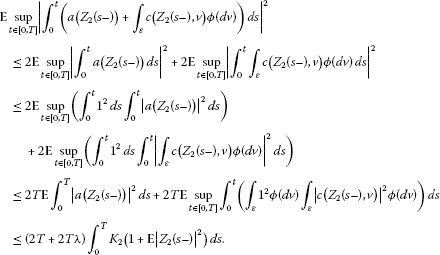(2.9)

Moreover, Doob’s martingale inequality (see ), the linear growth conditions (1.3) and Fubini’s theorem imply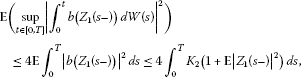(2.10)

and

$\begin{array}{rcl}\mathbf{E}\left(\underset{t\in \left[0,T\right]}{sup}{|{\int }_{0}^{t}{\int }_{\epsilon }c\left({Z}_{1}\left(s-\right),v\right){\stackrel{˜}{p}}_{\varphi }\left(dv×ds\right)|}^{2}\right)& \le & 4\mathbf{E}{\int }_{0}^{T}{\int }_{\epsilon }{|c\left({Z}_{1}\left(s-\right),v\right)|}^{2}\varphi \left(dv\right)\phantom{\rule{0.2em}{0ex}}ds\\ \le & 4{\int }_{0}^{T}{K}_{2}\left(1+\mathbf{E}{|{Z}_{1}\left(s-\right)|}^{2}\right)\phantom{\rule{0.2em}{0ex}}ds.\end{array}$
(2.11)

Thus, substituting (2.9), (2.10) and (2.11) into (2.8) on the interval $\left[0,T+1\right]$, we get the result (2.7) by taking

$\begin{array}{rcl}{C}_{2}& =& max\left\{4+\left(8T+8T\lambda \right){K}_{2}{C}_{1}T+32{K}_{2}{C}_{1}T,\\ \left(8T+8T\lambda \right){K}_{2}\left(1+{C}_{1}\right)T+32{K}_{2}\left(1+{C}_{1}\right)T\right\}.\end{array}$

□

The last lemma shows the close relation between the continuous-time implicit compensated Euler method (1.6) and its step functions ${Z}_{1}\left(t\right)$ and ${Z}_{2}\left(t\right)$.

Lemma 2.4 Under the conditions (1.3), there exist positive constants ${C}_{3}$ and ${C}_{4}$ independent of Δt such that for all $0<\mathrm{\Delta }t<\mathrm{\Delta }{t}_{0}$,

$\mathbf{E}\underset{t\in \left[0,T\right]}{sup}{|\overline{Y}\left(t\right)-{Z}_{1}\left(t\right)|}^{2}\le {C}_{3}\mathrm{\Delta }t\left(1+\mathbf{E}{|{x}_{0}|}^{2}\right),$
(2.12)

and

$\mathbf{E}\underset{t\in \left[0,T\right]}{sup}{|\overline{Y}\left(t\right)-{Z}_{2}\left(t\right)|}^{2}\le {C}_{4}\mathrm{\Delta }t\left(1+\mathbf{E}{|{x}_{0}|}^{2}\right).$
(2.13)

Proof It follows from (1.6) in $t\in \left[{t}_{n},{t}_{n+1}\right]\subseteq \left[0,T\right]$ that

$\begin{array}{rcl}\overline{Y}\left(t\right)-{Z}_{1}\left(t\right)& =& {Y}_{n}+{\int }_{{t}_{n}}^{t}\left(a\left({Z}_{2}\left(s-\right)\right)+{\int }_{\epsilon }c\left({Z}_{2}\left(s-\right),v\right)\varphi \left(dv\right)\right)\phantom{\rule{0.2em}{0ex}}ds+{\int }_{{t}_{n}}^{t}b\left({Z}_{1}\left(s-\right)\right)\phantom{\rule{0.2em}{0ex}}dW\left(s\right)\\ +{\int }_{{t}_{n}}^{t}{\int }_{\epsilon }c\left({Z}_{1}\left(s-\right),v\right){\stackrel{˜}{p}}_{\varphi }\left(dv×ds\right)-{Y}_{n}.\end{array}$

Using the inequality ${|{u}_{1}+{u}_{2}+{u}_{3}+{u}_{4}|}^{2}\le 4{|{u}_{1}|}^{2}+4{|{u}_{2}|}^{2}+4{|{u}_{3}|}^{2}+4{|{u}_{4}|}^{2}$ for ${u}_{1},{u}_{2},{u}_{3},{u}_{4}\in {\mathbf{R}}^{d}$, we have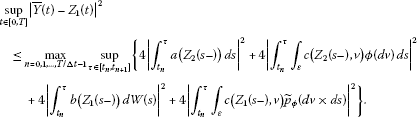(2.14)

Now, the Cauchy-Schwarz inequality gives

${|{\int }_{{t}_{n}}^{\tau }a\left({Z}_{2}\left(s-\right)\right)\phantom{\rule{0.2em}{0ex}}ds|}^{2}={\int }_{{t}_{n}}^{\tau }{1}^{2}\phantom{\rule{0.2em}{0ex}}ds{\int }_{{t}_{n}}^{\tau }{|a\left({Z}_{2}\left(s-\right)\right)|}^{2}\phantom{\rule{0.2em}{0ex}}ds\le \mathrm{\Delta }t{\int }_{{t}_{n}}^{{t}_{n+1}}{|a\left({Z}_{2}\left(s-\right)\right)|}^{2}\phantom{\rule{0.2em}{0ex}}ds,$
(2.15)

and

$\begin{array}{rcl}{|{\int }_{{t}_{n}}^{\tau }{\int }_{\epsilon }c\left({Z}_{2}\left(s-\right),v\right)\varphi \left(dv\right)\phantom{\rule{0.2em}{0ex}}ds|}^{2}& \le & {\int }_{{t}_{n}}^{\tau }{1}^{2}\phantom{\rule{0.2em}{0ex}}ds{\int }_{{t}_{n}}^{\tau }{|{\int }_{\epsilon }c\left({Z}_{2}\left(s-\right),v\right)\varphi \left(dv\right)|}^{2}\phantom{\rule{0.2em}{0ex}}ds\\ \le & \mathrm{\Delta }t{\int }_{{t}_{n}}^{{t}_{n+1}}{|{\int }_{\epsilon }c\left({Z}_{2}\left(s-\right),v\right)\varphi \left(dv\right)|}^{2}\phantom{\rule{0.2em}{0ex}}ds\\ \le & \mathrm{\Delta }t{\int }_{{t}_{n}}^{{t}_{n+1}}\left({\int }_{\epsilon }{1}^{2}\varphi \left(dv\right){\int }_{\epsilon }{|c\left({Z}_{2}\left(s-\right),v\right)|}^{2}\varphi \left(dv\right)\right)\phantom{\rule{0.2em}{0ex}}ds\\ =& \mathrm{\Delta }t\lambda {\int }_{{t}_{n}}^{{t}_{n+1}}{\int }_{\epsilon }{|c\left({Z}_{2}\left(s-\right),v\right)|}^{2}\varphi \left(dv\right)\phantom{\rule{0.2em}{0ex}}ds.\end{array}$
(2.16)

Moreover, Doob’s martingale inequality (see ) implies

$\mathbf{E}\left(\underset{\tau \in \left[{t}_{n},{t}_{n+1}\right]}{sup}{|{\int }_{{t}_{n}}^{\tau }b\left({Z}_{1}\left(s-\right)\right)\phantom{\rule{0.2em}{0ex}}dW\left(s\right)|}^{2}\right)\le 4\mathbf{E}{\int }_{{t}_{n}}^{{t}_{n+1}}{|b\left({Z}_{1}\left(s-\right)\right)|}^{2}\phantom{\rule{0.2em}{0ex}}ds,$
(2.17)

and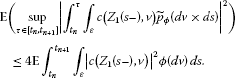(2.18)

Therefore, by substituting (2.15), (2.16), (2.17) and (2.18) into (2.14) and then applying the linear growth conditions (1.3) and Fubini’s theorem, we can obtain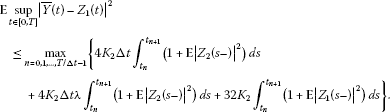Hence from (2.1), it follows that

$\begin{array}{rcl}\mathbf{E}\underset{t\in \left[0,T\right]}{sup}{|\overline{Y}\left(t\right)-{Z}_{1}\left(t\right)|}^{2}& \le & \mathrm{\Delta }t\left\{\left(1+{C}_{1}\right)\left(4{K}_{2}\mathrm{\Delta }{t}_{0}+4{K}_{2}\lambda \mathrm{\Delta }{t}_{0}+32{K}_{2}\right)\\ +{C}_{1}\left(4{K}_{2}\mathrm{\Delta }{t}_{0}+4{K}_{2}\lambda \mathrm{\Delta }{t}_{0}+32{K}_{2}\right)\mathbf{E}{|{x}_{0}|}^{2}\right\}\\ \le & {C}_{3}\mathrm{\Delta }t\left(1+\mathbf{E}{|{x}_{0}|}^{2}\right),\end{array}$

where ${C}_{3}=\left(1+{C}_{1}\right)\left(4{K}_{2}\mathrm{\Delta }{t}_{0}+4{K}_{2}\lambda \mathrm{\Delta }{t}_{0}+32{K}_{2}\right)$.

A similar analysis gives the result (2.13). □

Now, let us state the convergence of the implicit compensated Euler method by relying on the lemmas above.

Theorem 2.5 Under the Lipschitz conditions (1.2) and the linear growth conditions (1.3), the continuous implicit compensated Euler method (1.6) of the equation (1.1) satisfies

$\mathbf{E}\underset{t\in \left[0,T\right]}{sup}{|\overline{Y}\left(t\right)-x\left(t\right)|}^{2}\le {C}_{5}\left(1+\mathbf{E}{|{x}_{0}|}^{2}\right)\mathrm{\Delta }t,$
(2.19)

for all $0<\mathrm{\Delta }t<\mathrm{\Delta }{t}_{0}$, where ${C}_{5}$ is a constant independent of Δt.

Proof From (1.1) and (1.6), we can have

$\begin{array}{c}{|\overline{Y}\left(t\right)-x\left(t\right)|}^{2}\hfill \\ \phantom{\rule{1em}{0ex}}\le 4{|{\int }_{0}^{t}\left(a\left({Z}_{2}\left(s-\right)\right)-a\left(x\left(s-\right)\right)\right)\phantom{\rule{0.2em}{0ex}}ds|}^{2}+4{|{\int }_{0}^{t}\left(b\left({Z}_{1}\left(s-\right)\right)-b\left(x\left(s-\right)\right)\right)\phantom{\rule{0.2em}{0ex}}dW\left(s\right)|}^{2}\hfill \\ \phantom{\rule{2em}{0ex}}+4{|{\int }_{0}^{t}{\int }_{\epsilon }\left(c\left({Z}_{2}\left(s-\right),v\right)-c\left(x\left(s-\right),v\right)\right)\varphi \left(dv\right)\phantom{\rule{0.2em}{0ex}}ds|}^{2}\hfill \\ \phantom{\rule{2em}{0ex}}+4{|{\int }_{0}^{t}{\int }_{\epsilon }\left(c\left({Z}_{1}\left(s-\right),v\right)-c\left(x\left(s-\right),v\right)\right)\stackrel{˜}{p}\left(dv×ds\right)|}^{2},\hfill \end{array}$

where the inequality ${|{u}_{1}+{u}_{2}+{u}_{3}+{u}_{4}|}^{2}\le 4{|{u}_{1}|}^{2}+4{|{u}_{2}|}^{2}+4{|{u}_{3}|}^{2}+4{|{u}_{4}|}^{2}$ for ${u}_{1},{u}_{2},{u}_{3},{u}_{4}\in {\mathbf{R}}^{d}$ is used. Hence, by the Cauchy-Schwarz inequality and Doob’s martingale inequality, for any $0\le {t}_{0}\le T$, we obtain

$\begin{array}{c}\mathbf{E}\left(\underset{t\in \left[0,{t}_{0}\right]}{sup}{|\overline{Y}\left(t\right)-x\left(t\right)|}^{2}\right)\hfill \\ \phantom{\rule{1em}{0ex}}\le 4{t}_{0}\mathbf{E}{\int }_{0}^{{t}_{0}}{|a\left({Z}_{2}\left(s-\right)\right)-a\left(x\left(s-\right)\right)|}^{2}\phantom{\rule{0.2em}{0ex}}ds\hfill \\ \phantom{\rule{2em}{0ex}}+4{t}_{0}\lambda \mathbf{E}{\int }_{0}^{{t}_{0}}{\int }_{\epsilon }{|c\left({Z}_{2}\left(s-\right),v\right)-c\left(x\left(s-\right),v\right)|}^{2}\varphi \left(dv\right)\phantom{\rule{0.2em}{0ex}}ds\hfill \\ \phantom{\rule{2em}{0ex}}+16\mathbf{E}{\int }_{0}^{{t}_{0}}{|b\left({Z}_{1}\left(s-\right)\right)-b\left(x\left(s-\right)\right)|}^{2}\phantom{\rule{0.2em}{0ex}}ds\hfill \\ \phantom{\rule{2em}{0ex}}+16\mathbf{E}{\int }_{0}^{{t}_{0}}{\int }_{\epsilon }{|c\left({Z}_{1}\left(s-\right),v\right)-c\left(x\left(s-\right),v\right)|}^{2}\varphi \left(dv\right)\phantom{\rule{0.2em}{0ex}}ds.\hfill \end{array}$

Fubini’s theorem, the Lipschitz conditions (1.2) and the inequality ${|u+v|}^{2}\le 2{|u|}^{2}+2{|v|}^{2}$ then give

$\begin{array}{c}\mathbf{E}\left(\underset{t\in \left[0,{t}_{0}\right]}{sup}{|\overline{Y}\left(t\right)-x\left(t\right)|}^{2}\right)\hfill \\ \phantom{\rule{1em}{0ex}}\le 4T{K}_{1}{\int }_{0}^{{t}_{0}}\mathbf{E}{|{Z}_{2}\left(s-\right)-x\left(s-\right)|}^{2}\phantom{\rule{0.2em}{0ex}}ds+4T{K}_{1}\lambda {\int }_{0}^{{t}_{0}}\mathbf{E}{|{Z}_{2}\left(s-\right)-x\left(s-\right)|}^{2}\phantom{\rule{0.2em}{0ex}}ds\hfill \\ \phantom{\rule{2em}{0ex}}+32{K}_{1}{\int }_{0}^{{t}_{0}}\mathbf{E}{|{Z}_{1}\left(s-\right)-x\left(s-\right)|}^{2}\phantom{\rule{0.2em}{0ex}}ds\hfill \\ \phantom{\rule{1em}{0ex}}\le \left(8T{K}_{1}+8T{K}_{1}\lambda \right){\int }_{0}^{{t}_{0}}\mathbf{E}{|\overline{Y}\left(s\right)-{Z}_{2}\left(s-\right)|}^{2}\phantom{\rule{0.2em}{0ex}}ds+64{K}_{1}{\int }_{0}^{{t}_{0}}\mathbf{E}{|\overline{Y}\left(s\right)-{Z}_{1}\left(s-\right)|}^{2}\phantom{\rule{0.2em}{0ex}}ds\hfill \\ \phantom{\rule{2em}{0ex}}+\left(8T{K}_{1}+8T{K}_{1}\lambda +64{K}_{1}\right){\int }_{0}^{{t}_{0}}\mathbf{E}\underset{t\in \left[0,s\right]}{sup}{|\overline{Y}\left(t\right)-x\left(t-\right)|}^{2}\phantom{\rule{0.2em}{0ex}}ds.\hfill \end{array}$

Applying (2.12) and (2.13), we have

$\begin{array}{rcl}\mathbf{E}\left(\underset{t\in \left[0,{t}_{0}\right]}{sup}{|\overline{Y}\left(t\right)-x\left(t\right)|}^{2}\right)& \le & T\left(1+\mathbf{E}{|{x}_{0}|}^{2}\right)\left(8T{K}_{1}{C}_{4}+8T{K}_{1}\lambda {C}_{4}+64{K}_{1}{C}_{3}\right)\mathrm{\Delta }t\\ +\left(8T{K}_{1}+8T{K}_{1}\lambda +64{K}_{1}\right){\int }_{0}^{{t}_{0}}\mathbf{E}\underset{t\in \left[0,s\right]}{sup}{|\overline{Y}\left(t\right)-x\left(t-\right)|}^{2}\phantom{\rule{0.2em}{0ex}}ds.\end{array}$

The result (2.19) then follows from the continuous Gronwall inequality (see ). □

The theorem shows that the implicit compensated Euler method has strong order 0.5 under the conditions (1.2) and (1.3).

3 Mean-square stability

This section presents new results on the exponential mean-square stability for the equation (1.1) under the one-sided Lipschitz conditions in the following.

We assume that there exist constants ${\mu }_{1}$, ${\mu }_{2}$, ${\mu }_{3}$, ${\mu }_{4}$ such that $\mathrm{\forall }x,y\in {\mathbf{R}}^{d}$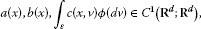(3.1)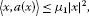(3.2)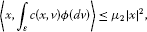(3.3)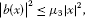(3.4)(3.5)

and

$a\left(0\right)=b\left(0\right)=c\left(0,0\right)=0.$
(3.6)

And so the equation (1.1) admits the trivial solution $x\left(t\right)\equiv 0$.

Since the numerical method (1.5) is implicit, the question of existence and uniqueness arises. And we solve this question in the following lemma.

Lemma 3.1 Under the conditions (3.1), (3.2) and (3.3), the equation for the implicit compensated Euler method (1.5) has a unique solution, with probability one, for all

$\left({\mu }_{1}+{\mu }_{2}\right)\mathrm{\Delta }t<1.$
(3.7)

Proof The required result is a special case of Theorem 14.2 in . □

In this paper, we consider the exponential mean-square stability of the trivial solution, which is defined according to [17, 19].

Definition 3.2 (see )

The equation (1.1) is said to be exponentially stable in the mean square if there is a pair of positive constants ${\alpha }_{1}$ and ${\alpha }_{2}$ such that for any initial data ${x}_{0}\in {L}_{{\mathcal{F}}_{0}}^{2}\left(\mathrm{\Omega };{\mathbf{R}}^{d}\right)$,

(3.8)

Definition 3.3 (see )

A numerical method applied to the equation (1.1) is said to be exponentially stable in the mean square, if there is a pair of positive constants ${\beta }_{1}$ and ${\beta }_{2}$ such that the numerical approximations ${Y}_{n}$ satisfy

$\mathbf{E}{|{Y}_{n}|}^{2}\le {\beta }_{1}\mathbf{E}{|{x}_{0}|}^{2}{e}^{-{\beta }_{2}\cdot n\mathrm{\Delta }t},\phantom{\rule{1em}{0ex}}n\in \mathbf{N},$
(3.9)

for initial data ${x}_{0}\in {L}_{{\mathcal{F}}_{0}}^{2}\left(\mathrm{\Omega };{\mathbf{R}}^{d}\right)$ and a given step size $\mathrm{\Delta }t>0$.

The result below shows the exponential mean-square stability of the equation (1.1).

Theorem 3.4 Under the conditions (3.1)-(3.6), if $\sigma <0$ in (3.11), then the equation (1.1) is exponentially stable in the mean square. More precisely, any analytical solution of the equation (1.1) with $\mathbf{E}{|{x}_{0}|}^{2}<\mathrm{\infty }$ satisfies

(3.10)

where

$\sigma :=2\left({\mu }_{1}+{\mu }_{2}\right)+{\mu }_{3}+{\mu }_{4}.$
(3.11)

Proof Using It$\stackrel{ˆ}{\mathrm{o}}$’s formula (see ) to $f\left(t,x\right)={|x\left(t\right)|}^{2}$, we have

$\begin{array}{rcl}d{|x\left(t\right)|}^{2}& =& \left(〈2x\left(t-\right),a\left(x\left(t-\right)\right)+{\int }_{\epsilon }c\left(x\left(t-\right),v\right)\varphi \left(dv\right)〉+{|b\left(x\left(t-\right)\right)|}^{2}\right)\phantom{\rule{0.2em}{0ex}}dt\\ +{\int }_{\epsilon }\left({|x\left(t-\right)+c\left(x\left(t-\right),v\right)|}^{2}-{|x\left(t-\right)|}^{2}-〈2x\left(t-\right),c\left(x\left(t-\right),v\right)〉\right)\varphi \left(dv\right)\phantom{\rule{0.2em}{0ex}}dt\\ +〈2x\left(t-\right),b\left(x\left(t-\right)\right)〉\phantom{\rule{0.2em}{0ex}}dW\left(t\right)+{\int }_{\epsilon }\left({|x\left(t-\right)+c\left(x\left(t-\right),v\right)|}^{2}-{|x\left(t-\right)|}^{2}\right){\stackrel{˜}{p}}_{\varphi }\left(dv×dt\right)\end{array}$

for all $t\ge 0$. Therefore, the conditions (3.1)-(3.5) give

$\begin{array}{rcl}d{|x\left(t\right)|}^{2}& \le & \left(\left(2{\mu }_{1}+2{\mu }_{2}+{\mu }_{3}+{\mu }_{4}\right){|x\left(t-\right)|}^{2}\right)\phantom{\rule{0.2em}{0ex}}dt+〈2x\left(t-\right),b\left(x\left(t-\right)\right)〉\phantom{\rule{0.2em}{0ex}}dW\left(t\right)\\ +{\int }_{\epsilon }\left({|x\left(t-\right)+c\left(x\left(t-\right),v\right)|}^{2}-{|x\left(t-\right)|}^{2}\right){\stackrel{˜}{p}}_{\varphi }\left(dv×dt\right).\end{array}$

Hence, we have

$\mathbf{E}{|x\left(t\right)|}^{2}\le \mathbf{E}{|{x}_{0}|}^{2}{e}^{\left(2{\mu }_{1}+2{\mu }_{2}+{\mu }_{3}+{\mu }_{4}\right)t},$

where the martingale properties of $dW\left(t\right)$ and ${\stackrel{˜}{p}}_{\varphi }\left(dv×dt\right)$ are used. □

The following lemma shows that the implicit compensated Euler (1.5) has a unique solution for all Δt under the condition $\sigma <0$ for the mean-square stability of the equation (1.1).

Lemma 3.5 Under the conditions (3.4) and (3.5), if $\sigma <0$ in (3.11), the implicit compensated Euler method (1.5) produces a well-defined unique solution.

Proof From the conditions (3.4) and (3.5), we get ${\mu }_{3}\ge 0$ and ${\mu }_{4}\ge 0$. Therefore, $\sigma <0$ in (3.10) can lead to $2\left({\mu }_{1}+{\mu }_{2}\right)<0$. Hence, for any $\mathrm{\Delta }t>0$, we have $\left({\mu }_{1}+{\mu }_{2}\right)\mathrm{\Delta }t<0<1$. So, according to Lemma 3.1, the equation for the implicit compensated Euler method (1.5) has a unique solution, with probability one, for all $\mathrm{\Delta }t>0$. □

In what follows, the theorem shows that as long as the equation (1.1) is exponentially stable in the mean square, the implicit compensated method (1.5) applied to (1.1) indeed shares the stability for any step size $\mathrm{\Delta }t>0$.

Theorem 3.6 Under the conditions (3.1)-(3.6), if $\sigma <0$ in (3.10) and (3.11), then for any step size $\mathrm{\Delta }t>0$ the implicit compensated Euler method (1.5) to the equation (1.1) is exponentially stable in the mean square, namely

$\mathbf{E}{|{Y}_{n}|}^{2}\le \mathbf{E}{|{x}_{0}|}^{2}{e}^{\beta \cdot n\mathrm{\Delta }t},\phantom{\rule{1em}{0ex}}n\in \mathbf{N},$
(3.12)

where $\beta \left(\mathrm{\Delta }t\right)=\sigma +O\left(\mathrm{\Delta }t\right)$, as $\mathrm{\Delta }t\to 0$.

Proof It follows from (1.5) that

$\begin{array}{c}{|{Y}_{n}-\left(a\left({Y}_{n}\right)+{\int }_{\epsilon }c\left({Y}_{n},v\right)\varphi \left(dv\right)\right)\mathrm{\Delta }t|}^{2}\hfill \\ \phantom{\rule{1em}{0ex}}={|{Y}_{n-1}+b\left({Y}_{n-1}\right)\mathrm{\Delta }{W}_{n-1}+{\int }_{{t}_{n-1}}^{{t}_{n}}{\int }_{\epsilon }c\left({Y}_{n-1},v\right){\stackrel{˜}{p}}_{\varphi }\left(dv×dt\right)|}^{2},\hfill \end{array}$

for any step size $\mathrm{\Delta }t>0$. Now, taking expectations and applying the martingale properties of $\mathrm{\Delta }{W}_{n-1}$ and ${\stackrel{˜}{p}}_{\varphi }\left(dv×dt\right)$, we get

$\begin{array}{rcl}\mathbf{E}{|{Y}_{n}|}^{2}& \le & 2\mathrm{\Delta }t\mathbf{E}〈{Y}_{n},a\left({Y}_{n}\right)+{\int }_{\epsilon }c\left({Y}_{n},v\right)\varphi \left(dv\right)〉+\mathbf{E}{|{Y}_{n-1}|}^{2}\\ +\mathrm{\Delta }t\mathbf{E}{|b\left({Y}_{n-1}\right)|}^{2}+\mathbf{E}{\int }_{{t}_{n-1}}^{{t}_{n}}{\int }_{\epsilon }{|c\left({Y}_{n-1},v\right)|}^{2}\varphi \left(dv\right)\phantom{\rule{0.2em}{0ex}}dt.\end{array}$

Under the conditions (3.2)-(3.5), we have

$\mathbf{E}{|{Y}_{n}|}^{2}\le 2\left({\mu }_{1}+{\mu }_{2}\right)\mathrm{\Delta }t\mathbf{E}{|{Y}_{n}|}^{2}+\left(1+{\mu }_{3}\mathrm{\Delta }t+{\mu }_{4}\mathrm{\Delta }t\right)\mathbf{E}{|{Y}_{n-1}|}^{2}.$

Since the conditions of (3.10) and (3.11) in Theorem 3.4 give

$1-2\left({\mu }_{1}+{\mu }_{2}\right)\mathrm{\Delta }t>0,$

and

$0<\frac{1+{\mu }_{3}\mathrm{\Delta }t+{\mu }_{4}\mathrm{\Delta }t}{1-2\left({\mu }_{1}+{\mu }_{2}\right)\mathrm{\Delta }t}<1,$

we get

$\mathbf{E}{|{Y}_{n}|}^{2}\le \mathbf{E}{|{x}_{0}|}^{2}{e}^{\beta \left(\mathrm{\Delta }t\right)\cdot n\mathrm{\Delta }t},$

where

□

4 Numerical experiments

This section presents several numerical experiments that demonstrate the results about convergence and stability in Section 2 and Section 3.

We consider the following equation:

$dx\left(t\right)=ax\left(t-\right)\phantom{\rule{0.2em}{0ex}}dt+bx\left(t-\right)\phantom{\rule{0.2em}{0ex}}dW\left(t\right)+{\int }_{\epsilon }cx\left(t-\right)v{p}_{\varphi }\left(dv×dt\right),\phantom{\rule{1em}{0ex}}t>0,$
(4.1)

with $x\left(0\right)$, where $d=m=r=1$. The compensated measure of the Poisson random measure ${p}_{\varphi }\left(dv×dt\right)$ is given by $\varphi \left(dv\right)\phantom{\rule{0.2em}{0ex}}dt=\lambda f\left(v\right)\phantom{\rule{0.2em}{0ex}}dv\phantom{\rule{0.2em}{0ex}}dt$, and

$f\left(v\right)=\frac{1}{\sqrt{2\pi }v}exp\left(-\frac{{\left(lnv\right)}^{2}}{2}\right),\phantom{\rule{1em}{0ex}}0\le v<\mathrm{\infty },$

is the density function of a lognormal random variable. We simulate $W\left(t\right)$, ${p}_{\varphi }\left(t\right)$ and ${\xi }_{i}$ which are from independent sources of randomness, mainly according to [2, 20]. Here three examples are given as

$\begin{array}{ll}\mathrm{I}:& a=-2,\phantom{\rule{2em}{0ex}}b=1,\phantom{\rule{2em}{0ex}}c=-0.1,\phantom{\rule{2em}{0ex}}\lambda =2,\\ \mathrm{II}:& a=-3,\phantom{\rule{2em}{0ex}}b=1,\phantom{\rule{2em}{0ex}}c=-0.1,\phantom{\rule{2em}{0ex}}\lambda =2,\end{array}$

and

$\mathrm{III}:\phantom{\rule{1em}{0ex}}a=0,\phantom{\rule{2em}{0ex}}b=0,\phantom{\rule{2em}{0ex}}c=-0.1,\phantom{\rule{2em}{0ex}}\lambda =2.$

In Figure 1, the convergence of the implicit compensated Euler method (1.7) to I, II and III is described. In this numerical test, we focus on the error at the endpoint $T=1$, and the endpoint error is denoted as $\mathbf{E}|x\left(T\right)-Y\left(T\right)|$. The expectation is estimated by averaging $M=1\text{,}000$ sample paths over $\left[0,1\right]$, and for each path, the implicit compensated method is applied with four different step sizes $\mathrm{\Delta }t={2}^{p}\delta t$ for $1\le p\le 4$, $\delta t=T/{2}^{12}$. The solid line shows a log-log plot of the expectation $\mathbf{E}|x\left(T\right)-Y\left(T\right)|$ against Δt. For reference, a dashed line of slope 0.5 is added. We can see that the computational result is consistent with a strong order equal to 0.5.

To observe the performance of the stability of the implicit compensated Euler method (1.7) to (4.1), we compare the method with the Euler method (see ) and the drift-implicit Euler method (see ) for an increasing step size Δt in Figure 2, Figure 3, Figure 4 and Figure 5. Here, $\mathbf{E}{|{Y}_{n}|}^{2}$ denotes the expectation of the numerical approximations. The expectation is estimated by averaging $M=50\text{,}000$ sample paths, that is to say, ${\omega }_{i}:1\le i\le 50\text{,}000$, $\mathbf{E}{|{Y}_{n}|}^{2}=1/50\text{,}000{\sum }_{i=1}^{50\text{,}000}{|{Y}_{n}\left({\omega }_{i}\right)|}^{2}$. According to Theorem 3.4, I, II and III are exponentially stable in the mean square.

From Figure 2, for a small step size $\mathrm{\Delta }t=1/{2}^{5}$, the three numerical methods to I are mean-square stable and behave similarly. Moreover, for a step size $\mathrm{\Delta }t=1/{2}^{2}$, slight difference appears among the three methods. However, from Figure 3, for a larger step size $\mathrm{\Delta }t=1$, there is observable instability of the Euler method and drift-implicit Euler method while the implicit compensated Euler method remains stable for I. Therefore, our numerical results show that for the parameter values used here, the Euler method and drift-implicit Euler method are stable in the mean square only for restricted step sizes. It is interesting to observe that even for a very large step size $\mathrm{\Delta }t=60$, the implicit compensated Euler method is still stable in the mean square for I and II in Figure 4. And in Figure 5, pure jump example III is described.

So, we can think that the numerical experiments are consistent with our results in Section 3, that is to say, the implicit Euler compensated method performs better stability than current numerical methods of order 0.5.

References

1. 1.

Schönbucher PJ: Credit Derivatives Pricing Models: Models, Pricing and Implementation. Wiley, Chichester; 2003:86–103.

2. 2.

Cont R, Tankov P Financ. Math. Ser. In Financial Modelling with Jump Processes. Chapman & Hall/CRC, Boca Raton; 2004.

3. 3.

Merton RC: Option pricing when underlying stock returns are discontinuous. J. Financ. Econom. 1976, 2: 125–144.

4. 4.

Platen E: An approximation method for a class of Itô processes with jump component. Liet. Mat. Rink. 1982, 22(2):124–136.

5. 5.

Bruti-Liberati, N, Platen, E: On the strong approximation of jump-diffusion processes. Technical report, Quantitative Finance Research Papers 157, University of Technology, Sydney (2005)

6. 6.

Bruti-Liberati N, Platen E: Strong approximations of stochastic differential equations with jumps. J. Comput. Appl. Math. 2007, 205: 982–1001. 10.1016/j.cam.2006.03.040

7. 7.

Bruti-Liberati N, Platen E: Approximation of jump diffusions in finance and economics. Comput. Econ. 2007, 29: 283–312. 10.1007/s10614-006-9066-y

8. 8.

Mao W: Convergence of numerical solutions for variable delay differential equations driven by Poisson random jump measure. Appl. Math. Comput. 2009, 212: 409–417. 10.1016/j.amc.2009.02.041

9. 9.

Buckwar E, Riedler MG: Runge-Kutta methods for jump-diffusion differential equations. J. Comput. Appl. Math. 2011. doi:10.1016/j.cam.2011.08.001

10. 10.

Liu XQ, Li CW: Weak approximations and extrapolations of stochastic differential equations with jumps. SIAM J. Numer. Anal. 2000, 37(6):1747–1767. 10.1137/S0036142998344512

11. 11.

Mordecki E, Szepessy A, Tempone R, Zouraris GE: Adaptive weak approximation of diffusions with jumps. SIAM J. Numer. Anal. 2008, 46(4):1732–1768. 10.1137/060669632

12. 12.

Li CW, Dong Z, Situ R: Almost sure stability of linear stochastic differential equations with jumps. Probab. Theory Relat. Fields 2002, 123: 121–155. 10.1007/s004400200198

13. 13.

Swishchuk AV, Kazmerchuk YI: Stability of stochastic differential delay Itô’s equations with Poisson jumps and with Markovian switchings. Application to financial models. Teor. Imovir. Mater. Stat. 2001, 64: 141–151.

14. 14.

Higham DJ, Kloeden PE: Numerical methods for nonlinear stochastic differential equations with jumps. Numer. Math. 2005, 101(1):101–119. 10.1007/s00211-005-0611-8

15. 15.

Ikeda N, Watanabe S: Stochastic Differential Equations and Diffusion Processes. 2nd edition. North-Holland, Amsterdam; 1989.

16. 16.

Øksendal B, Sulem A Universitext. In Applied Stochastic Control of Jump-Diffusions. Springer, Berlin; 2005.

17. 17.

Mao XR, Yuan CG: Stochastic Differential Equations with Markovian Switching. Imperial College Press, London; 2006:17–200.

18. 18.

Hairer E, Wanner G: Solving Ordinary Differential Equations II: Stiff and Differential-Algebraic Problems. 2nd edition. Springer, Berlin; 1996:215–217.

19. 19.

Liu MZ, Cao WR, Fan ZC: Convergence and stability of the semi-implicit Euler method for a linear stochastic differential delay equation. J. Comput. Appl. Math. 2004, 170: 255–268. 10.1016/j.cam.2004.01.040

20. 20.

Higham DJ: An algorithmic introduction to numerical simulation of stochastic differential equations. SIAM Rev. 2001, 43(3):525–546. 10.1137/S0036144500378302

Acknowledgements

The financial support from the National Natural Science Foundation of China (No. 11071050) is gratefully acknowledged.

Author information

Authors

Corresponding author

Correspondence to Minghui Song.

Competing interests

The authors declare that they have no competing interests.

Authors’ contributions

The authors have equal contributions to each part of this article. All the authors read and approved the final manuscript.

Authors’ original submitted files for images

Below are the links to the authors’ original submitted files for images.

Rights and permissions

Reprints and Permissions

Song, M., Yu, H. Convergence and stability of implicit compensated Euler method for stochastic differential equations with Poisson random measure. Adv Differ Equ 2012, 214 (2012). https://doi.org/10.1186/1687-1847-2012-214

• Accepted:

• Published:

• DOI: https://doi.org/10.1186/1687-1847-2012-214

Keywords

• stochastic differential equations
• Poisson random measure
• convergence
• exponential mean-square stability